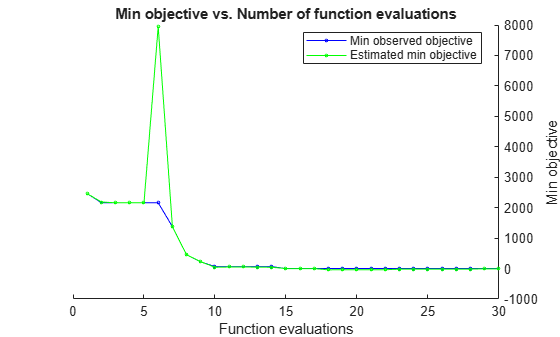# resume

Resume a Bayesian optimization

## Syntax

``newresults = resume(results,Name,Value)``

## Description

example

````newresults = resume(results,Name,Value)` resumes the optimization that produced `results` with additional options specified by one or more `Name,Value` pair arguments.```

## Examples

collapse all

This example shows how to resume a Bayesian optimization. The optimization is for a deterministic function known as Rosenbrock's function, which is a well-known test case for nonlinear optimization. The function has a global minimum value of `0` at the point `[1,1]`.

Create two real variables bounded by `-5` and `5`.

```x1 = optimizableVariable('x1',[-5,5]); x2 = optimizableVariable('x2',[-5,5]); vars = [x1,x2]; ```

Create the objective function.

```function f = rosenbrocks(x) f = 100*(x.x2 - x.x1^2)^2 + (1 - x.x1)^2; ```
```fun = @rosenbrocks; ```

For reproducibility, set the random seed, and set the acquisition function to `'expected-improvement-plus'` in the optimization.

```rng default results = bayesopt(fun,vars,'Verbose',0,... 'AcquisitionFunctionName','expected-improvement-plus'); ```View the best point found and the best modeled objective.

```results.XAtMinObjective results.MinEstimatedObjective ```
```ans = 1x2 table x1 x2 ______ ______ 1.5256 2.3109 ans = -16.1075 ```

The best point is somewhat close to the optimum, but the function model is inaccurate. Resume the optimization for 30 more points (a total of 60 points), this time telling the optimizer that the objective function is deterministic.

```newresults = resume(results,'IsObjectiveDeterministic',true,'MaxObjectiveEvaluations',30); newresults.XAtMinObjective newresults.MinEstimatedObjective ```
```ans = 1x2 table x1 x2 ______ ______ 1.0077 1.0144 ans = 0.0014 ```The objective function model is much closer to the true function this time. The best point is closer to the true optimum.

## Input Arguments

collapse all

Bayesian optimization results, specified as a `BayesianOptimization` object.

### Name-Value Arguments

Specify optional pairs of arguments as `Name1=Value1,...,NameN=ValueN`, where `Name` is the argument name and `Value` is the corresponding value. Name-value arguments must appear after other arguments, but the order of the pairs does not matter.

Before R2021a, use commas to separate each name and value, and enclose `Name` in quotes.

Example: `resume(results,'MaxObjectiveEvaluations',60)`

You can use any name-value pair accepted by `bayesopt` except for those beginning with `Initial`. See the `bayesopt` Input Arguments.

Note

The `MaxObjectiveEvaluations` and `MaxTime` name-value pairs mean additional time or evaluations, above the numbers stored in `results`. So, for example, the default number of evaluations is `30` in addition to the original specification.

Additionally, you can use the following name-value pair.

Modify variable, specified as an `OptimizableVariable` object.

You can change only the following properties of a variable in an optimization.

• `Range` of real or integer variables. For example,

```xvar = optimizableVariable('x',[-10,10]); % Modify the range: xvar.Range = [1,5];```
• `Type` between `'integer'` and `'real'`. For example,

`xvar.Type = 'integer';`
• `Transform` of real or integer variables between `'log'` and `'none'`. For example,

`xvar.Transform = 'log';`

## Output Arguments

collapse all

Optimization results, returned as a `BayesianOptimization` object.

## Version History

Introduced in R2016b